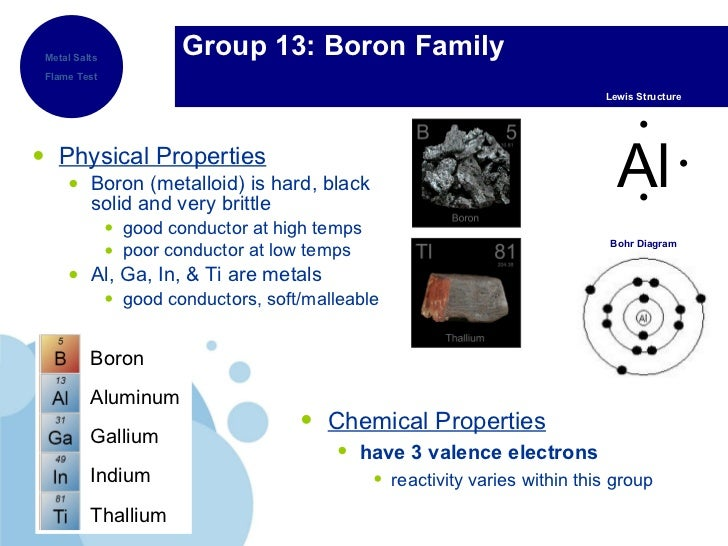# what is the difference between the reactivity and abundance of elements in Group 3A through Group 8A?

May 26, 2017List down the elements in Group 8A

$\text{Group 8A (or VIIIA)}$ of the periodic table are the noble gases or inert gases: helium (He), neon (Ne), argon (Ar), krypton (Kr), xenon (Xe), and radon (Rn). The name comes from the fact that these elements are virtually unreactive towards other elements or
compounds.

Well I've listed the abundance of each element in Group 8A

Argon = 0.96%(in the atmosphere) + 3.5×10^(−6)"kg/kg"(in the crust)
Helium =  8×10^(−9)"kg/kg"(in the crust)
Neon = 5×10^(−9)"kg/kg"(in the crust)
Krypton = 1×10^(−10)"kg/kg"(in the crust)
Xenon = 3×10^(-11)"kg/kg"(in the crust)Source :Crust: CRC Handbook

List down the elements in Group 3A

Boron = 2.8×10^(-6)"kg/kg"(in the crust)
Aluminium = (8.4100×10^(-2)"kg)/kg(in the crust)
There's also 2.2% aluminium in the mantle
Gallium = 1.9×10^(-5)"kg/kg"(in the crust)
Indium = 2.5×10^(-7)"kg/kg"(in the crust)
Thallium = 8.5×10^(-7)"kg/kg"(in the crust)I've listed the abundance of each element in Group 3A

So we can see that the abundance of the elements in Group 3A is more than that of abundance of elements in Group 8A

And since noble gases are non-reactive the elements in Group 3A are more reactive than the elements in Group 8A